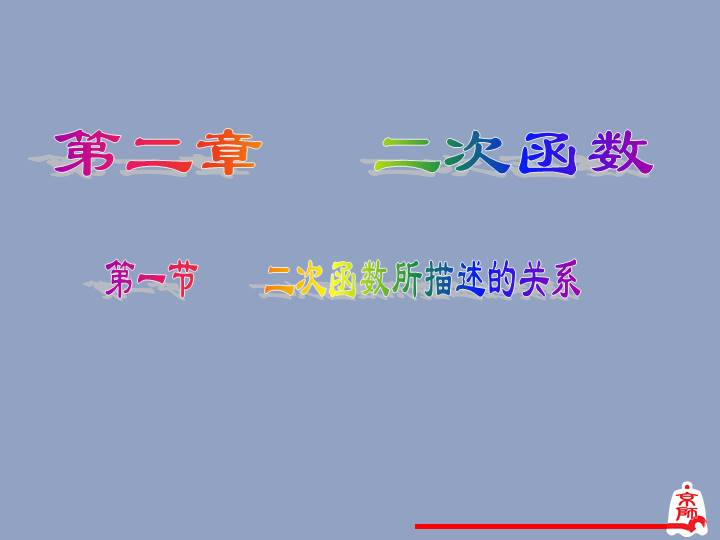# 第二章：二次函数所描述的关系3、什么叫做反比例函数？
4、函数有哪些表示方法？

(1)问题中有哪些变量? 其中哪些是自变量? 哪些是因变量?

(100+x)棵 (600-5x)个 某果园有100棵橙子树，每一棵树平均结600个橙子。现准备多种一些橙子树以提高产量，但是如果多种树，那么树之间的距离和每一棵树所接受的阳光就会减少．根据经验估计，每多种一棵树，平均每棵树就会少结5个橙子。

y= (100+x) (600-5x)
=-5x2+100x+60000

y=(100+x)(600-5x)=-5x2+100x+60000.

60375 60420 60455 60480 60495 60500 60495 60480 60455 60420 60375 y=-5x2+100x+60000,

y=100(x+1)2=100x2+200x+100.

y=-5x2+100x+60000, y=100x2+200x+100.

y=-5x2+100x+60000, y=100x2+200x+100.

(2)等式的右边最高次数为2,可以没有一次项 和常数项,但不能没有二次项.

1.定义：一般地,形如y=ax2+bx+c(a, b,c是常数,a≠0)的函数叫做x的二次函数. y=ax2+bx+c(a,b,c是常数,a≠0) 的几种不同表示形式: (1)y=ax2 --------- (a≠0,b=0,c=0,). (2)y=ax2+c ------ (a≠0,b=0,c≠0). (3)y=ax2+bx ---- (a≠0,b≠0,c=0).

1.下列函数中,哪些是二次函数？

(3) s=3-2t2.
(5) v=πr2.
（是） （是） （是） （不是） （不是） 大胆尝试，练一练！
2.用总长为60m的篱笆围成矩形场地，场地面积S(m2)与矩形一边长a(m)之间的关系是什么？是函数关系吗？是哪一种函数？

4.如果函数y=(k-3) +kx+1是二次函数,则k的值一定是______ .
0 3.如果函数y= +kx+1是二次函数, 则k的值一定是______ .
0或3 开拓创新 试一试
5.圆的半径是4cm,假设半径增加xcm时, 圆的面积增加ycm2. （1）写出y与x之间的函数关系表达式； （2）当圆的半径分别增加1cm, , 2cm时,圆的面积增加多少？

1.定义：一般地,形如y=ax2+bx+c(a, b,c是常数,a≠0)的函数叫做x的二次函数. y=ax2+bx+c(a,b,c是常数,a≠0) 的几种不同表示形式: (1)y=ax2 --------- (a≠0,b=0,c=0,). (2)y=ax2+c ------ (a≠0,b=0,c≠0). (3)y=ax2+bx ---- (a≠0,b≠0,c=0).

2.定义的实质是：ax2+bx+c是整 式,自变量x的最高次数是二次.